# Diode Current Equation

## What is the Diode Current Equation?

The diode current equation expresses the relationship between the current flowing through the diode as a function of the voltage applied across it. Mathematically the diode current equation can be expressed as: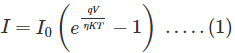Where,

• I is the current flowing through the diode
• I0 is the dark saturation current,
• q is the charge on the electron,
• V is the voltage applied across the diode,
• η is the (exponential) ideality factor.
•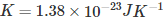is the Boltzmann constant
• T is the absolute temperature in Kelvin.

In this equation, two parameters require to be discussed in quite detail.

They are I0, the Dark Saturation Current, and η, the (exponential) Ideality Factor.

Dark saturation current (I0) indicates the leakage current density flowing through the diode in the absence of light (hence, ‘dark’).

This parameter is the characteristic of the diode under consideration and indicates the amount of recombination which occurs within it.

That is, I0 will be larger for a diode in which recombination rate is higher and vice versa. Further, its value is also seen to be directly proportional to the absolute temperature and inversely proportional to the material quality.

η, the (exponential) Ideality Factor

Ideality factor (η) indicates the nearness with which the considered diode behaves with respect to the ideal diode.

That is, if the diode under consideration behaves exactly as that of an ideal diode, then η will be 1. Its value increases from 1 as the difference between the behaviors of the ideal diode and diode under consideration increases: greater is the deviation, greater is the value of η.

The value of η is typically considered to be 1 for germanium diodes and 2 for silicon diodes.

However, its exact value for the given diode depends on various factors like electron drift, diffusion, carrier recombination which occurs within the depletion region, its doping level, manufacturing technique and the purity of its materials.

In addition, its value is also seen to vary with the value of current and voltage levels. Nevertheless, in most of the cases, its value is found to be within the range 1 to 2.

In forward biased condition, there will a large amount of current flow through the diode. Thus the diode current equation (equation 1) becomes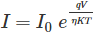On the other hand, if the diode is reverse biased, then the exponential term in equation (1) becomes negligible. Thus we have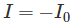Now let us examine the mode the diode current equation takes its form when we have the diode operating at room temperature. In this case, T = 300 K, also,and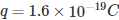. Thus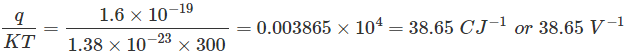By reciprocating, one gets, 25.87 mV which is called thermal voltage. Thus the diode equation at room temperature becomes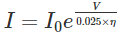Want To Learn Faster? 🎓
Get electrical articles delivered to your inbox every week.
No credit card required—it’s 100% free.